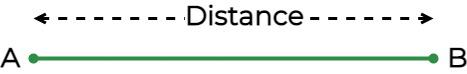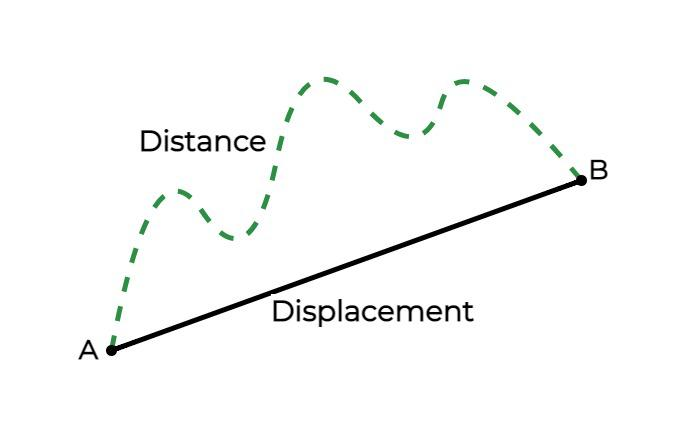# Distance and Displacement

Distance and Displacement are two crucial terms of Mechanics that may seem the same but have different meanings and definitions. Distance is a measure of “How much path is covered by an object in motion?” While Displacement is the measure of “How much path is covered by the object in a particular direction?” Hence, the major difference between distance and displacement is that distance is a scalar quantity and displacement is a vector quantity. Despite this difference, both quantities have some similarities. The unit of distance and displacement is meter (m).

In this article, we will learn about Distance Definition in Physics, Displacement Definition in physics, Distance Formula, Displacement Formula, Distance and Displacement Examples, and others in detail.

## What is Distance?

Distance is the total path covered by the object in motion, irrespective of the direction of the path. Distance is known as a scalar quantity as only the path length covered is measured and the direction of motion is not considered. The distance can never be zero or negative, it can only be positive.

Distance gives detailed information about the entire path covered during motion. Distance is represented as ‘d’ and it is not represented with an arrow as the direction is not involved. The distance between points A and B is shown in the image added below.## Distance Formula

Mathematically, Distance can be defined as “Distance is the Total length of Path Covered from One Point to Another by an object.”

We know that,

Speed = Distance / Time

Therefore using the above formula we get the formula for the distance when the speed and time of any motion is given is,

Distance = Speed × Time

d = s × t

where,

• d is the Distance Covered Object
• s is the Speed
• t is the Time Taken

## What is Displacement?

Displacement is defined as the total change in the object’s position along with the direction of motion. Displacement is known to be vector quantity as along with the magnitude of the changed position, the direction of the motion is also taken into account. Displacement can be positive, negative, or zero.

Displacement does not give detailed information on the entire path length, the initial and the final position is required for the calculation of the displacement. Displacement is represented as ‘S’ and an arrow is used to indicate that displacement is a vector quantity. Displacement always has a straight-lined path as the initial and final points are directly connected to find the displacement in the image added below the distance and displacement between points A and B.## Displacement Formula

Mathematically, the displacement is defined as, the “Minimum distance between two points.” The formula used to find the displacement is,

∆x = xf – x0

where,

• xf is the Final Position of Object
• x0 is the Starting Position of Object
• ∆x is the Displacement of Object

## Distance Vs Displacement

Distance is the length of the path taken by an object whereas displacement is the simply the distance between the initial point and final point of the object. The differences between Distance and Displacement are shown in the table added below,

Distance

Displacement

Distance is the total path covered by the object in motion, irrespective of the direction of the path. Displacement is defined as the total change in the object’s position along with the direction of motion.
Distance is represented by the letter d. Displacement is represented by the letter s.
Distance is a Scalar Quantity. Displacement is a Vector Quantity.
When Distance is calculated, only the length of the path is considered, ignoring its direction. When Displacement is calculated, both the length of the path and the direction of the object are considered.
The formula to calculate the distance (d) is,
Speed × Time
The formula to calculate the Displacement (s) is,
Velocity × Time
The distance can only have positive values. However, displacement can be positive, negative, or zero.

## Similarities Between Distance and Displacement

Despite a number of differences between them, both distance and displacement have quite similarities as well, some of which are listed below:

• SI unit of both Distance and Displacement is meter (m).
• Both distance and displacement depend on the initial and final points for measurement.
• When the direction is not considered both are equal in magnitude, in most cases.
• The dimensional formula for Distance and Displacement is the same.

## Examples on Distance and Displacement Formula

Example 1: A particle has covered a distance of 1000 meters and a displacement of 200 meters in 1 min 40 sec. Find the Speed and Velocity of the particle during motion.

Solution:

Time Taken = 1 min 40 sec = 100 sec

Speed = Distance/ Time

Speed = 1000/ 100 = 10 m/sec

Velocity = Displacement/ Time

Velocity = 200/100 = 2 m/sec

Example 2: The speed and the velocity of a car are given as 5 m/sec, and the time taken in the whole journey is 5 minutes, what are the observations drawn from that information?

Solution:

Time taken in Whole Journey = 5 mins = 5 × 60= 300 sec

Speed = 5 m/sec

Distance = 5 × 300 = 1500 m = 1.5 km

Velocity = 5 m/sec

Displacement = 5× 300= 1500 m = 1.5 km

Observation: The distance and the displacement are both equal since the speed and the velocity of the car is equal. Therefore, it can be said that the car has traveled only in a straight direction.

Example 3: A boy decided to take a walk around his town in the evening, he started from his home and traveled approximately 1500 meters in 30 minutes. He finally came back to his home. Find the values of the following quantities,

• Speed
• Displacement
• Velocity

Solution:

Given

• Distance= 1500 m
• Time= 30 minutes= 30× 60 seconds= 1800 s

Speed = Distance/ Time

Speed= 1500/1800 = 0.833 m/sec

Displacement = 0 m (as the boy finally came back to his house, so the initial and the final point coincided).

Velocity = Displacement/ Time = 0/ 1800 = 0 m/sec

## Distance and Displacement-FAQs

### 1. What is the Distance Definition?

The total length covered by the object from point one to another point is the distance covered.

### 2. What is the Displacement Definition?

Displacement is defined as the net difference between the length in the initial position and the final position of the object.

### 3. What is the Differences between Distance and Displacement?

Difference between distance and displacement,

 Distance Displacement Distance is the total path length It is the length between initial and final points. Distance has no direction Displacement has direction Distance gives detailed info about path length Displacement does not give detailed information about the path length.

### 4. Is it Possible to Have a Negative Distance?

No, distance can never be negative. Distance is always positive. However, displacement can be negative.

### 5. Can Displacement be Zero?

Yes, displacement can be zero when the initial position and final position of the object is equal.

### 6. When does the Distance and Magnitude of Displacement equal?

The distance and magnitude of displacement become equal when the motion is in a fixed direction or the motion is unidirectional.

### 7. What is an Example of Distance and Displacement?

• Example of the Distance is, suppose a boy moves from his house to his school which is at a distance of 5 km.
• Example of the Displacement is, suppose the boy returns from his school to his house tracing the same path then the displacement of the boy is zero.

Whether you're preparing for your first job interview or aiming to upskill in this ever-evolving tech landscape, GeeksforGeeks Courses are your key to success. We provide top-quality content at affordable prices, all geared towards accelerating your growth in a time-bound manner. Join the millions we've already empowered, and we're here to do the same for you. Don't miss out - check it out now!

Previous
Next